# 【C++、数据结构】封装map和set（用红黑树实现）

### 文章目录

• 📖 前言
• 1. 如何复用同一棵红黑树⚡
• 1.1 🌀 修改后结点的定义：
• 2. 模拟实现中何实现数据比较大小🌟
• 3. 红黑树迭代器的实现🔥
• 3.1 💥红黑树begin（）和 end（）的定义
• 3.2 💥 operator* 和 operator->
• 3.3 💥 operator++ 和 operator- -
• 3.4 💥 operator== 和 operator!=
• 4. 封装map和set⭕

# 1. 如何复用同一棵红黑树⚡

• map和set都是用的同一棵红黑树复用的吗
• 或者这两个容器各自使用一棵红黑树吗• map和set都是在复用同一棵红黑树
• 它们实现的都是Key_value模型

• 对于set而言，T就是RBTree<K, K>
• 对于map而言，T就是RBTree<K, pair<K, V>>

• 首先，答案肯定是不能的
• 那么原因又是什么呢？• map和set都是用了，Key_value模型
• set中的K是K，V也是K
• map中的K是K，V是pair<K，V>
• 并且模板参数中第一个K都不能省

### 1.1 🌀 修改后结点的定义：

``````enum Colour
{RED,BLACK,
};//上层维度的一个泛型
template<class T>
struct RBTreeNode
{RBTreeNode<T>* _left;RBTreeNode<T>* _right;RBTreeNode<T>* _parent;T _data;	//数据Colour _col;RBTreeNode(const T& data): _data(data), _left(nullptr), _right(nullptr), _parent(nullptr), _col(RED){}
};
``````

# 2. 模拟实现中何实现数据比较大小🌟

• 上述提到，在模拟实现中，map和set我们复用同一棵红黑树的时候都是用的是Kye_value的结构
• 但是红黑树中的数据比较又是Key值的比较，而现在我们用的则是pair的比较
• 虽然编译上是可以通过但是真的就是我们所想要的吗？

pair比较大小：• 根据map和set的需求不同
• 我们在红黑树中新引入了一个模板参数map中的仿函数：set中的仿函数：``````//红黑树的实现
//KeyOfT --> 支持取出T对象中key的仿函数
template<class K, class T, class KeyOfT>
class RBTree
{typedef RBTreeNode<T> Node;
public:typedef __RBTreeIterator<T, T&, T*> iterator;typedef __RBTreeIterator<T, const T&, const T*> const_iterator;//构造 拷贝构造 赋值 和析构 跟搜索树实现方式是一样的//迭代器中序遍历，要找最左结点iterator Begin(){Node* subLeft = _root;while (subLeft && subLeft->_left){subLeft = subLeft->_left;}//树的迭代器用结点的指针就可以构造return iterator(subLeft);}iterator End(){return iterator(nullptr);}const_iterator Begin() const{Node* subLeft = _root;while (subLeft && subLeft->_left){subLeft = subLeft->_left;}//树的迭代器用结点的指针就可以构造return const_iterator(subLeft);}const_iterator End() const{return const_iterator(nullptr);}pair<iterator, bool> Insert(const T& data){//1、搜索树的规则插入//2、看是否违反平衡规则，如果违反就需要处理：旋转if (_root == nullptr){_root = new Node(data);_root->_col = BLACK; //根节点是黑色return make_pair(iterator(_root), true);}KeyOfT kot;Node* parent = nullptr;Node* cur = _root;while (cur){if (kot(cur->_data) < kot(data)){parent = cur;cur = cur->_right;}else if (kot(cur->_data) > kot(data)){parent = cur;cur = cur->_left;}else{return make_pair(iterator(cur), false);}}//找到符合规则的位置之后再插入cur = new Node(data); Node* newnode = cur;cur->_col = RED;if (kot(parent->_data) < kot(data)){parent->_right = cur;}else{parent->_left = cur;}//三叉链的链接 -- 链上父节点cur->_parent = parent;//存在连续红色结点while (parent && parent->_col == RED){//理论而言，祖父是一定存在的，父亲存在且是红不可能是根（根一定是黑的）Node* grandfather = parent->_parent;assert(grandfather);if (grandfather->_left == parent){Node* uncle = grandfather->_right;//情况一：（叔叔存在且为红）if (uncle && uncle->_col == RED){//祖父和叔叔变成黑色parent->_col = uncle->_col = BLACK;grandfather->_col = RED;//继续往上处理cur = grandfather;parent = cur->_parent;}//情况二：（叔叔不存在 or 叔叔存在且为黑）else{//单旋//	   g//	 p// cif (cur == parent->_left){RotateR(grandfather);parent->_col = BLACK;grandfather->_col = RED;}//双旋//    g//  p//    celse{RotateL(parent);RotateR(grandfather);cur->_col = BLACK;grandfather->_col = RED;}break;}}//无论父亲和叔叔是左是右都是一样的//grandfather->_right == parent;else{Node* uncle = grandfather->_left;//情况一：if (uncle && uncle->_col == RED){//祖父和叔叔变成黑色parent->_col = uncle->_col = BLACK;grandfather->_col = RED;//继续往上处理cur = grandfather;parent = cur->_parent;}else{//单旋// g//   p//     cif (cur == parent->_right){RotateL(grandfather);parent->_col = BLACK;grandfather->_col = RED;}//双旋//  g//    p//  celse{RotateR(parent);RotateL(grandfather);cur->_col = BLACK;grandfather->_col = RED;}break;}}}//父亲为空就出循环，将根节点设置成黑色_root->_col = BLACK;return make_pair(iterator(newnode), true);}
}
``````

# 3. 红黑树迭代器的实现🔥

### 3.1 💥红黑树begin（）和 end（）的定义• 因为是二叉搜索树，为了更有顺序，所以我们采取的是中序遍历。
• 那么中序遍历Begin（）就应该是最左结点
• 我们实现的版本中End（）定义为空是（nullptr）``````template<class T, class Ref, class Ptr>
struct __RBTreeIterator
{typedef RBTreeNode<T> Node;Node* _node;typedef __RBTreeIterator<T, Ref, Ptr> Self;__RBTreeIterator(Node* node):_node(node){}Ref operator*(){return _node->_data;}Ptr operator->(){return &_node->_data;}Self& operator++(){if (_node->_right == nullptr){//找祖先里面，孩子是父亲左的那个Node* cur = _node;Node* parent = cur->_parent;while (parent && parent->_right == cur){cur = cur->_parent;parent = parent->_parent;}_node = parent;}else{//右子树的最左结点Node* subLeft = _node->_right;while (subLeft->_left){subLeft = subLeft->_left;}//左为空_node = subLeft;}return *this;}Self& operator--(){if (_node->_left == nullptr){//找祖先里面，孩子是父亲Node* cur = _node;Node* parent = cur->_parent;while (parent && cur == parent->_left){cur = cur->_parent;parent = parent->_parent;}_node = parent;}else{//左子树的最右结点Node* subRight = _node->_left;while (subRight->_right){subRight = subRight->_right;}_node = subRight;}return *this;}Self operator++(int){Self tmp(*this);++(*this);return tmp;}Self operator--(int){Self tmp(*this);--(*this);return tmp;}bool operator!=(const Self& s) const{return _node != s._node;}bool operator==(const Self& s) const{return _node == s._node;}
};
``````
• T:数据
• Ref：引用
• Ptr：指针
• Sef：iterator本身

### 3.2 💥 operator* 和 operator->### 3.3 💥 operator++ 和 operator- -it走到哪，说明哪个结点已经访问过了，接下来我们的操作不是递归，但是胜似递归

• 跟着中序遍历的方式，it肯定是从5->6->7->8
• 很显然这时我们只需要看当前位置右子树是否是空

1. 右子树为空：找孩子是父亲的左的那个祖先节点，否则继续往上走，直到空（nullptr）
2. 右子树为非空：找右子树的最左节点• 很显然这时我们只需要看当前位置左子树是否是空

1. 左子树为空：找孩子是父亲的右的那个祖先节点，否则继续往上走，直到空（nullptr）
2. 左子树为非空：找左子树的最右节点### 3.4 💥 operator== 和 operator!=``````iterator Find(const K& key)
{Node* cur = _root;KeyOfT kot;while (cur){if (kot(cur->_data) < key){cur = cur->_right;}else if (kot(cur->_data) > key){cur - cur->_left;}else{return iterator(cur);}return End();}
}
``````

# 4. 封装map和set⭕

map的封装：

``````template<class K, class V>
class map
{//定义一个内部类struct MapKeyOfT{const K& operator()(const pair<K, V>& kv)//operator() 可以像函数一样去使用{return kv.first;}};public:typedef typename RBTree<K, pair<K, V>, MapKeyOfT>::iterator iterator;typedef typename RBTree<K, pair<K, V>, MapKeyOfT>::const_iterator const_iterator;iterator begin(){return _t.Begin();}iterator end(){return _t.End();}pair<iterator, bool> insert(const pair<K, V>& kv){return _t.Insert(kv);}V& operator[](const K& key){pair<iterator, bool> ret = insert(make_pair(key, V()));return ret.first->second;}
private:RBTree<K, pair<K, V>, MapKeyOfT> _t;
};
``````

``````template<class K>
class set
{struct SetKeyOfT{const K& operator()(const K& key)//operator() 可以像函数一样去使用{return key;}};
public://加上typename告诉编译器这是个类型，类模板实例化了再去取typedef typename RBTree<K, K, SetKeyOfT>::const_iterator iterator;typedef typename RBTree<K, K, SetKeyOfT>::const_iterator const_iterator;iterator begin() const{return _t.Begin();}iterator end() const{return _t.End();}pair<iterator, bool> insert(const K& key){//pair<typename RBTree<K, K, SetKeyOfT>::iterator, bool> ret = _t.Insert(key);auto ret = _t.Insert(key);return pair<iterator, bool>(iterator(ret.first._node), ret.second);}iterator find(const K& key){return _t.Find(key);}private:RBTree<K, K, SetKeyOfT> _t;
};
``````

### 相关文章：## 【C++、数据结构】封装map和set（用红黑树实现）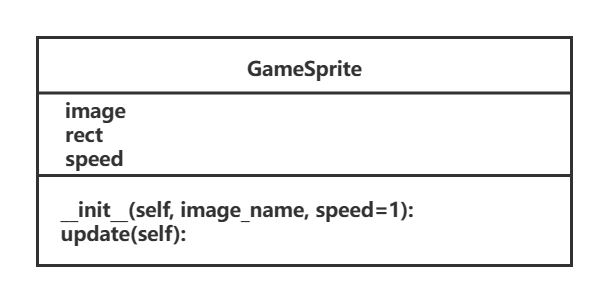## Python-项目实战--飞机大战-pygame快速入门(3)

4.理解精灵和精灵组4.1精灵和精灵组在刚刚完成的案例中&#xff0c;图像加载、位置变化、绘制图像都需要程序员编写代码分别处理为了简化开发步骤&#xff0c;pygame提供了两个类pygame.sprite.Sprite --存储图像数据 image和位置rect的对象pygame.sprite.Group注意&#xff1a…...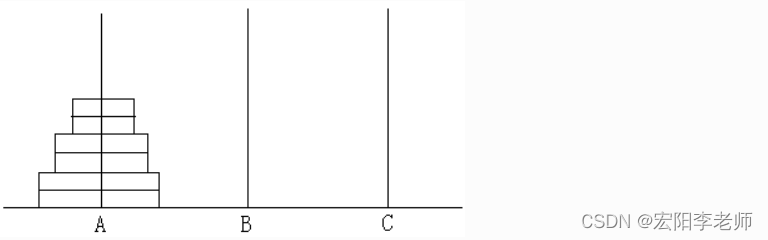## 【2007NOIP普及组】T4.Hanoi双塔问题 试题解析

【2007NOIP普及组】T4.Hanoi双塔问题 试题解析 时间限制: 1000 ms 内存限制: 65536 KB 【题目描述】 给定A,B,C三根足够长的细柱，在A柱上放有2n个中间有空的圆盘，共有n个不同的尺寸，每个尺寸都有两个相同的圆盘，注意这两个圆盘是不加区分的(下图为n=3的情形）。现要…...## Camtasia2023免费屏幕录像软件支持MP4/AVI/WMV等多种格式## MySQL中的事务(隔离性详解)

1.什么是事务事务是指逻辑上的一组操作,组成这组操作的各个单元,要么全部成功,要么全部失败(通俗的说一组SQL语句,要么全部执行成功,一条语句出错则全部出粗);在不同的环境中,都可以有事务,对应在数据库中,就是数据库事务.2.为什么使用事务事务的核心逻辑就是多条SQL语句要么全…...## iPhone苹果手机Apple id帐号如何永久性注销删除数据？## CSDN第26期周赛赛后总结（第一次AK）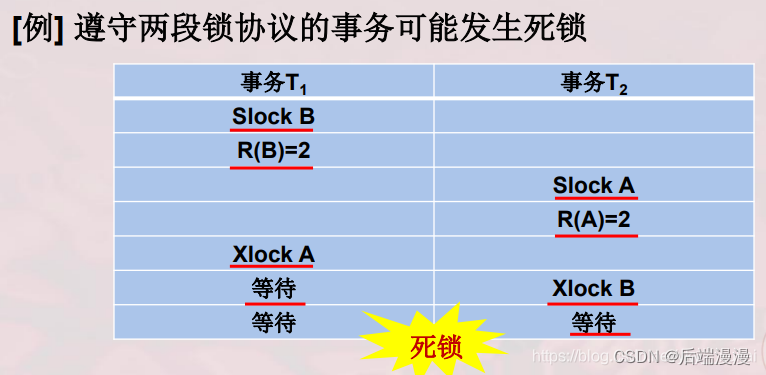## MySQL面试题：MySQL锁机制## 带你三分钟了解网络用语之计算机网络概述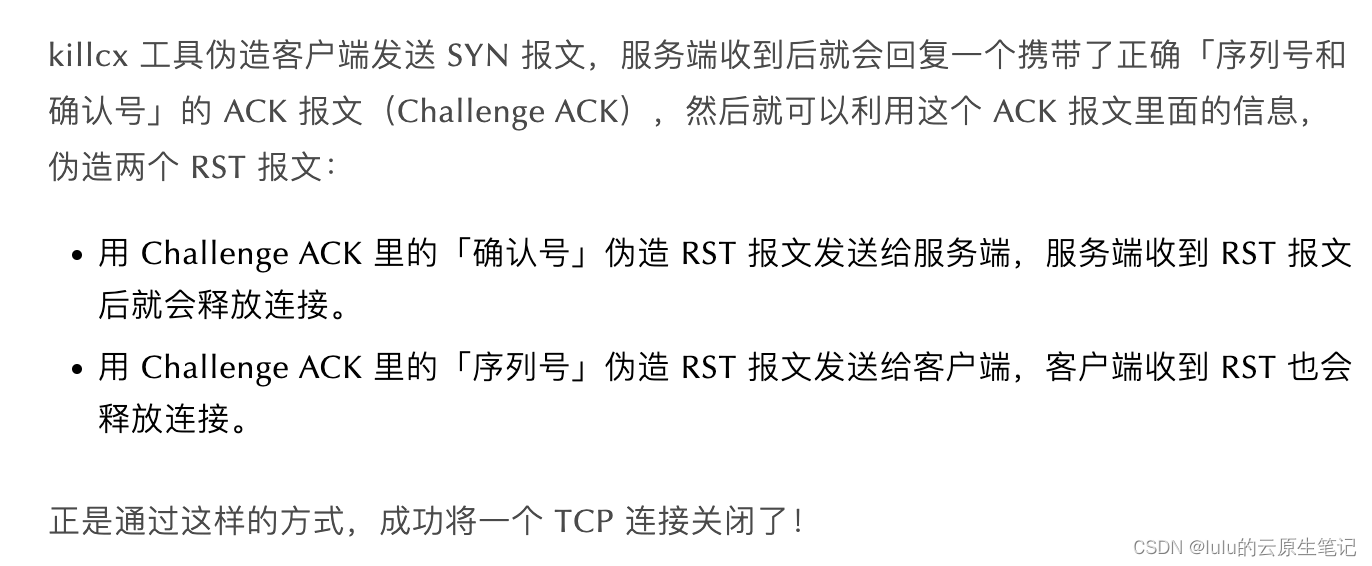## 【博客613】tcp重置防火墙原理：构造RST报文来终结非法活跃连接

tcp重置防火墙原理&#xff1a;构造RST报文来终结非法活跃连接 1、场景 如何关闭一个 TCP 连接&#xff1f; 可能大家第一反应是「杀掉进程」不就行了吗&#xff1f; 是的&#xff0c;这个是最粗暴的方式&#xff0c;杀掉客户端进程和服务端进程影响的范围会有所不同&#…...## (2023Q2模拟题JAVA)华为OD机试 - 最多提取子串数目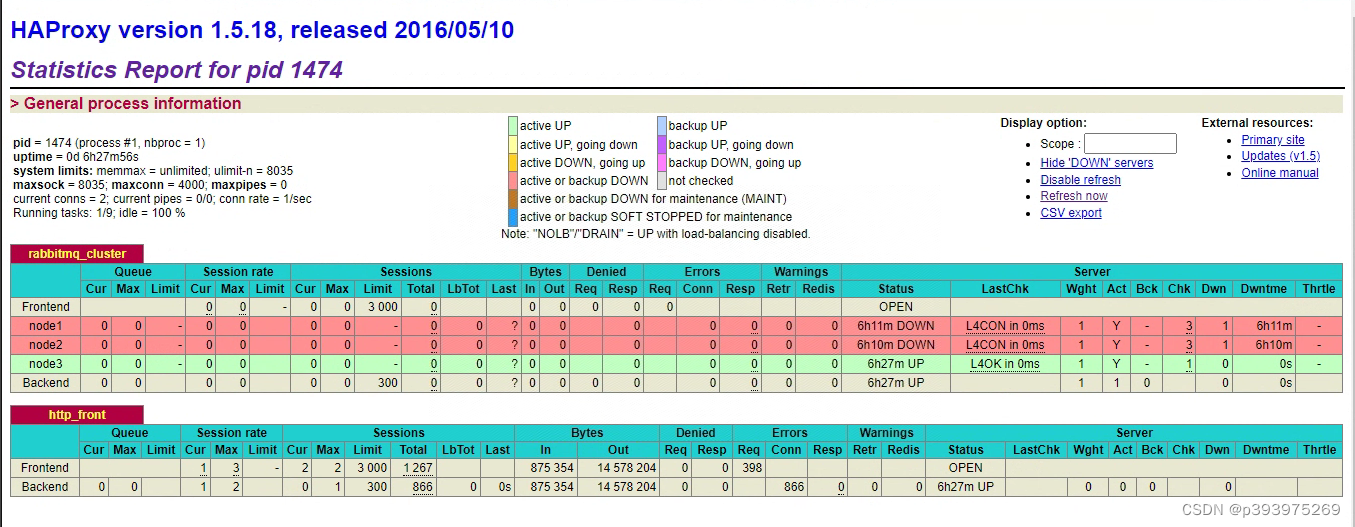## docker-compose部署rabbitmq集群

1、集群分类 RabbitMQ的是基于Erlang语言编写&#xff0c;而Erlang又是一个面向并发的语言&#xff0c;天然支持集群模式。 RabbitMQ的集群以下分类&#xff1a; 标准集群&#xff1a;是一种分布式集群&#xff0c;将队列分散到集群的各个节点&#xff0c;从而提高整个集群的并…...## 如何实现手势识别功能？## Cocos2d-x 屏幕适配新解(比较全面比较详细)## 如何优雅的管理游戏资源

[叶落归根]&#xff1a; http://blog.leafsoar.com/archives/2013/11-27.html 在游戏的开发过程中&#xff0c;前期的规划 往往比 后期的“优化”更为重要&#xff01;比如多分辨率适配&#xff0c;如果前期没有规划好&#xff0c;可能导致的情况是&#xff0c;画面只在当前测试…...## 【SQL开发实战技巧】系列（三十）：数仓报表场景☞树形(分层)查询如何排序？以及如何在树形查询中正确的使用where条件## 修建灌木顺子日期## ARM LCD 编程实战## 被大厂废掉的年轻人